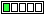All about flooble | fun stuff | Get a free chatterbox | Free JavaScript | Avatarsperplexus dot infoProve irrationality (Posted on 2011-08-07)Prove that lg(3) is irrational.

Rem: lg(m)=t implies 10^t=m

 See The Solution Submitted by Ady TZIDON Rating: 5.0000 (2 votes)Comments: ( Back to comment list | You must be logged in to post comments.)Proof| Comment 1 of 2
Suppose log(3) is rational. Then, for some integers x and y, log(3)=x/y. Here is what we get.
ylog(3)=x
10^(ylog(3))=10^x
3^y=10^x
However, all powers of 3 are odd, and powers of 10 are even. Therefore, log(3) is irrational.

 Posted by Math Man on 2011-08-07 13:47:55Please log in:

 Search: Search body:
Forums (0)